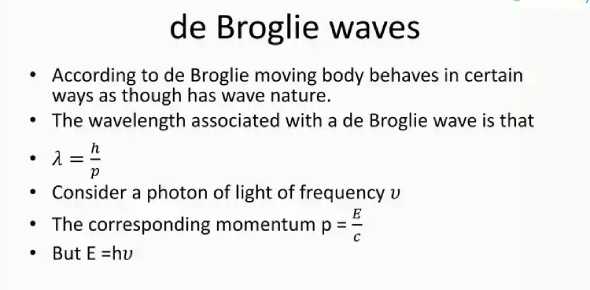# De Broglie Wave Quiz: Physics Test!

6 Questions | Attempts: 1977Settings.

• 1.
Complete the following statement: According to the de Broglie relation, the wavelength of a "matter" wave is inversely proportional to
• A.

Planck's constant.

• B.

The mass of the particle.

• C.

The momentum of the particle.

• D.

The frequency of the wave.

• E.

The speed of the particle.

• 2.
What is the de Broglie wavelength of an electron (m = 9.11 ´ 10-31 kg) in a 5.0 ´ 103-volt X-ray tube?
• A.

0.007 nm

• B.

0.014 nm

• C.

0.017 nm

• D.

0.028 nm

• E.

0.034 nm

• 3.
Determine the de Broglie wavelength of a neutron (m = 1.67 ´ 10-27 kg) which has a speed of 5.0 m/s.
• A.

79 nm

• B.

162 nm

• C.

395 nm

• D.

529 nm

• E.

1975 nm

• 4.
The de Broglie wavelength of an electron (m = 9.11 ´ 10-31 kg) is 1.2 ´ 10-10 m. Determine the kinetic energy of the electron.
• A.

1.5 x 10^-15 J

• B.

1.6 x 10^-16 J

• C.

1.7 x 10^-17 J

• D.

1.8 x 10^-18 J

• E.

1.9 x 10^-19 J

• 5.
What happens to the de Broglie wavelength of an electron if its momentum is doubled?
• A.

The wavelength decreases by a factor of 4.

• B.

The wavelength increases by a factor of 4.

• C.

The wavelength increases by a factor of 3.

• D.

The wavelength increases by a factor of 2.

• E.

The wavelength decreases by a factor of 2.

• 6.
The Hubble Space Telescope has an orbital speed of 7.56 ´ 103 m/s and a mass of 11,600 kg. What is the de Broglie wavelength of the telescope?
• A.

8.77 x 10^7 m

• B.

5.81 x 10^-26 m

• C.

6.63 x 10^-34 m

• D.

3.78 x 10^-40 m

• E.

7.56 x 10^-42 m

## Related TopicsBack to top
×

Wait!
Here's an interesting quiz for you.